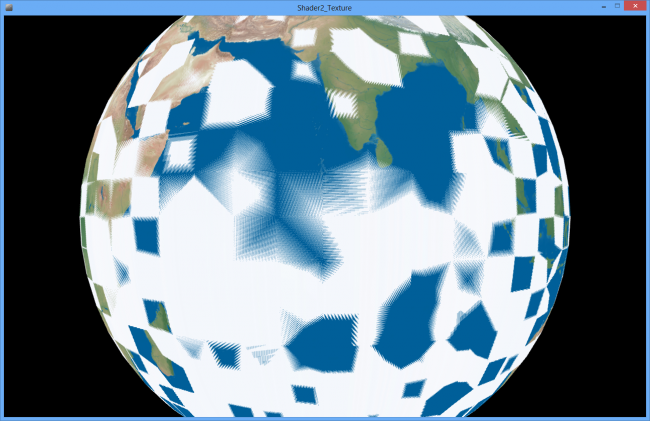#### Howdy, Stranger!

We are about to switch to a new forum software. Until then we have removed the registration on this forum.

# Problems drawing a sphere

edited January 2014

This demo takes the processing demo on drawing textures and attempts to create a sphere. I successfully get the right shape, but there are blue and white quads being drawn on top of the texture and I don't know why.

I have attached a screenshot. You can see the earth, but on top of it are lots of blue and white quads in various patterns.

I figured out that the reason it wasn't working was that I was using a QUAD_STRIP and the strips don't connect. So I tried to create multiple QUAD_STRIPS, one for each band around the earth. That doesn't work because PShape seems to support only a single one. I could use QUADS and write each intervening point twice, like this:

B C C E E G

A D D F F H ....

But since that still doesn't solve the problem of writing the poles, I would like to know what I should do to add this code into PShape.

In addition, when I tried to use quads, I think the code no longer worked with the GLSL. Here are the GLSL files:

``````#ifdef GL_ES
precision mediump float;
precision mediump int;
#endif

uniform sampler2D texture;

varying vec4 vertColor;
varying vec4 vertTexCoord;

void main() {
gl_FragColor = texture2D(texture, vertTexCoord.st) * vertColor;
}

uniform mat4 transform;
uniform mat4 texMatrix;

attribute vec4 vertex;
attribute vec4 color;
attribute vec2 texCoord;

varying vec4 vertColor;
varying vec4 vertTexCoord;

void main() {
gl_Position = transform * vertex;

vertColor = color;
vertTexCoord = texMatrix * vec4(texCoord, 1.0, 1.0);
}
``````

Here's the processing source code

``````void setup() {
size(640, 360, P3D);
can = createSphere(200, 64, label);
}

void draw() {
background(0);
translate(width/2, height/2);
rotateX(-PI/2);
rotateZ(angle);
shape(can);
angle += 0.01;
}

PShape createSphere(float r, int detail, PImage tex) {
textureMode(NORMAL);
PShape sh = createShape();
sh.noStroke();
sh.texture(tex);
final float dA = TWO_PI / detail; // change in angle

// process the sphere one band at a time
// going from almost south pole to almost north
// poles must be handled separately
float theta2 = -PI/2+dA;
float SHIFT = PI/2;
float z2 = sin(theta2); // height off equator
float rxyUpper = cos(theta2); // closer to equator
for (int i = 1; i < detail; i++) {
float theta1 = theta2;
theta2 = theta1 + dA;
float z1 = z2;
z2 = sin(theta2);
float rxyLower = rxyUpper;
rxyUpper = cos(theta2); // radius in xy plane
for (int j = 0; j <= detail; j++) {
float phi = j * dA; //longitude in radians
float xLower = rxyLower * cos(phi);
float yLower = rxyLower * sin(phi);
float xUpper = rxyUpper * cos(phi);
float yUpper = rxyUpper * sin(phi);
float u = phi/TWO_PI;
sh.normal(xLower, yLower, z1);
sh.vertex(r*xLower, r*yLower, r*z1, u,(theta1+SHIFT)/PI);
sh.normal(xUpper, yUpper, z2);
sh.vertex(r*xUpper, r*yUpper, r*z2, u,(theta2+SHIFT)/PI);
}
}
sh.endShape();
return sh;
}
``````Tagged:

• The main problem in your code was that you were increasing the theta angle (which goes from -pi to pi) by the step corresponding to the phi angle.

Regarding using multiple quad strips: you can create a separate shape for each strip, and then group them together using a group shape.

Also, you were assigning PI/2 to a variable named SHIFT, which is not recommended since Processing uses the name SHIFT to identify the shift key.

With these corrections, a working version of the sketch could be:

``````PImage label;
PShape earth;
float angle;

void setup() {
size(640, 360, P3D);
earth = createSphere(150, 64, label);
}

void draw() {
background(0);
translate(width/2, height/2);
rotateX(-PI/2);
rotateZ(angle);
shape(earth);
angle += 0.01;
}

PShape createSphere(float r, int detail, PImage tex) {
textureMode(NORMAL);
PShape gr = createShape(GROUP);

float dphi = TWO_PI / detail; // change in phi angle
float dtheta = PI / detail; // change in theta angle

// process the sphere one band at a time
// going from almost south pole to almost north
// poles must be handled separately
float theta2 = -PI/2+dtheta;
float z2 = sin(theta2); // height off equator
float rxyUpper = cos(theta2); // closer to equator
for (int i = 1; i < detail; i++) {
float theta1 = theta2;
theta2 = theta1 + dtheta;
float z1 = z2;
z2 = sin(theta2);
float rxyLower = rxyUpper;
rxyUpper = cos(theta2); // radius in xy plane

PShape sh = createShape();
sh.noStroke();
sh.texture(tex);
for (int j = 0; j <= detail; j++) {
float phi = j * dphi; //longitude in radians
float xLower = rxyLower * cos(phi);
float yLower = rxyLower * sin(phi);
float xUpper = rxyUpper * cos(phi);
float yUpper = rxyUpper * sin(phi);
float u = phi/TWO_PI;
sh.normal(xLower, yLower, z1);
sh.vertex(r*xLower, r*yLower, r*z1, u,(theta1+HALF_PI)/PI);
sh.normal(xUpper, yUpper, z2);
sh.vertex(r*xUpper, r*yUpper, r*z2, u,(theta2+HALF_PI)/PI);
}
sh.endShape();